Create a new printable
Answer key also includes questions
Answer key only gives the answers
No answer keyMath Worksheets
Time

Sample - Click above to make a new math worksheet (PDF).
 Name _____________________________Date ___________________
Hour
Write the time two ways.

1.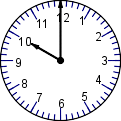___ o'clock___ : ___
2.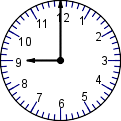___ o'clock___ : ___
3.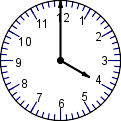___ o'clock___ : ___
4.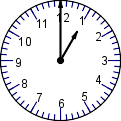___ o'clock___ : ___
5.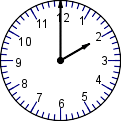___ o'clock___ : ___
6.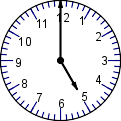___ o'clock___ : ___
7.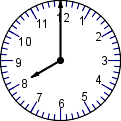___ o'clock___ : ___
8.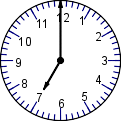___ o'clock___ : ___
9.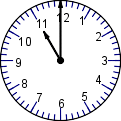___ o'clock___ : ___
 10 * This is a pre-made sheet.Use the link at the top of the page for a printable page.
11.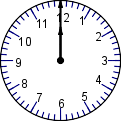___ o'clock___ : ___
12.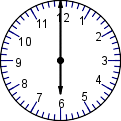___ o'clock___ : ___

 Answer Key

Sample
This is only a sample worksheet.

Create a new printable
Answer key also includes questions
Answer key only gives the answers
No answer keyMath Worksheets
Time